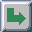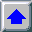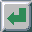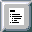Next: Human Vision Up: Color Science Basics Previous: Photometry

# Colorimetry

Colorimetry is the science of measuring colors. Although each of us can perceive colors slightly differently, the CIE has defined a standard observer. A set of standard conditions for performing color measuring experiments has also been proposed by CIE. A number of color matching experiments have been performed under these standardized conditions. Color matching experiments consists of choosing three particular light sources, that emit light on the white screen, where three projections overlap and form an additive mixture. On the other side of the screen a target color is projected, and an observer tries to match the target light by altering the intensities of the three light sources. The weights of light sources are in the range [-1,1]. Negative weights are allowed, as it is not possible to match all colors using only positive weights. A negative weight does not mean subtracting color from the additive mixture, but rather adding this color to the target color. After many experiments using light sources of the wavelengths red=700 nm, green=546.1 nm and blue is 435.8 nm [WySt82] color matching curves as shown in figure 2.2 were proposed by CIE.Figure 2.2: The r, g, and b color-matching curves [WySt82]Figure 2.3: The x, y, and z color-matching curves [WySt82]

As it was inconvenient to have negative values in the matching functions CIE proposed a linear transformation of matching functions resulting in CIE x, y and z matching functions, as shown in figure 2.3. Note that there are no negative values in these matching functions.

Now if the surface reflectance, and the light source distribution are known, their product defines color as, and the weights XYZ can be found using the following equations:The weights, X, Y and Z define a color in the CIE XYZ space. Note that it is possible that two objects with different spectral reflectance, under certain illuminantion, appear the same, i.e. have the same CIE XYZ values. The CIE XYZ is a 3D linear color space, and it is quite awkward to work in it directly. It is common to project this space to the X+Y+Z=1 plane. The result is a 2D space known as the CIE chromaticity diagram. The coordinates in this space are usually called x and y and they are derived from XYZ using the following equations:As the z component bears no additional information, it is often omitted. Note that since xy space is just a projection of the 3D XYZ space, each point in xy corresponds to many points in the original space. Actually the missing information is luminance Y. Color is usually described by xyY coordinates, where x and y determine the chromaticity and Y the lightness component of color. Figure 2.4 shows the CIE xy chromaticity diagram.Figure 2.4: CIE xy chromaticity diagram

Chromaticity diagrams can give us a lot of useful information on a particular color. The horseshoe shaped curve represents the whole spectrum. The straight line connecting the lowest wavelength blue and the highest wavelength red is called the ``purple line'' and does not represent spectral colors. The white point lies somewhere inside the diagram, depending on the light source used (e.g. D65 light source is defined to simulate day-light and has x=0.312727 and y=0.329024). If a line is drawn through the white point and a particular xy color, then the ratio between this point to white point distance, and the spectral line to the white point distance gives us the color saturation. If the color is close to the spectral line its saturation is high. The dominant wavelength which determines a color's hue is determined by the intersection of the line with the spectral line. An interesting property of the xy chromaticity diagram is that all possible mixtures of colorsandare given by the straight line connecting these two points. It is clear that all the possible mixtures of the three colors,andthen lie inside the triangle determined with those three points. Now, it is clear that the color gamut of any display device using three primaries (like a standard CRT monitor) is only a subset of all visible colors.

In spite of all the useful characteristics of the CIE xy chromaticity diagram, it lacks one very important characteristic. Namely, if the distance between any arbitrary two points is the same as the distance between an other point pair, the perceived distance will not be the same. In the worst case, if the perceived distances are the same, actual distances can differ as much as 20 times. In order to correct this, researchers are trying to find a perceptually uniform color space. It has, unfortunately, still not been found. CIE proposed two alternatives as improvements compared with CIE xyY space. These are CIE LUV and CIE LAB. Although they are referred to as perceptually uniform color spaces by some authors, they are not. Just for comparison, two perceptually equally distant color pairs, can differ in the CIE LUV distance as much as 4 times. This is a significant improvement compared to 20 times by original space, but it is still not perfect. Conversions between CIE XYZ and CIE LUV are defined with the formulas:whereThe distance between two colors in the CIE LUV space can be computed using CIE LUV color difference formula:Next: Human Vision Up: Color Science Basics Previous: Photometry

matkovic@cg.tuwien.ac.at